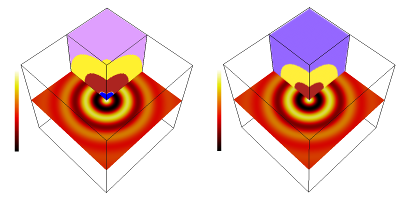## Parallel computation of 3D Morse-Smale complexes.Nithin Shivashankar and Vijay Natarajan.
Computer Graphics Forum (EuroVis 2012), 31(3), 2012, 965-974.

### Abstract

The Morse-Smale complex is a topological structure that captures the behavior of the gradient of a scalar function on a manifold. This paper discusses scalable techniques to compute the Morse-Smale complex of scalar functions defined on large three-dimensional structured grids. Computing the Morse-Smale complex of three-dimensional domains is challenging as compared to two-dimensional domains because of the non-trivial structure introduced by the two types of saddle criticalities. We present a parallel shared-memory algorithm to compute the Morse- Smale complex based on Forman's discrete Morse theory. The algorithm achieves scalability via synergistic use of the CPU and the GPU. We first prove that the discrete gradient on the domain can be computed independently for each cell and hence can be implemented on the GPU. Second, we describe a two-step graph traversal algorithm to compute the 1-saddle-2-saddle connections efficiently and in parallel on the CPU. Simultaneously, the extrema-saddle connections are computed using a tree traversal algorithm on the GPU.